# Concept of Lines Symmetry

#### Topics

• Figures with one Line of Symmetry
• Figures with two Line of Symmetry
• Figures with more Line of Symmetry

## Definition

• Line Symmetry: A figure has line symmetry if a line can be drawn dividing the figure into two symmetrical parts. The line is called a line of symmetry.

## Notes

### Line Symmetry:

A figure has line symmetry if a line can be drawn dividing the figure into two symmetrical parts. The line is called a line of symmetry.

A figure can have any number of lines of symmetry passing through it. Some can have one, two, or even multiple lines of symmetry.

#### 1. Figures with one Line of Symmetry:

A figure has line symmetry if there is a line about which the figure may be folded so that the two parts of the figure will coincide.

#### 2. Figures with two Lines of Symmetry:

Example of two lines of symmetry: A rectangle.

• Take a rectangular sheet (like a post-card).
• Fold it once lengthwise so that one-half fits exactly over the other half.
• Open it up now and again fold on its width in the same way.
• After opening it, we get two lines of symmetry of the rectangular sheet.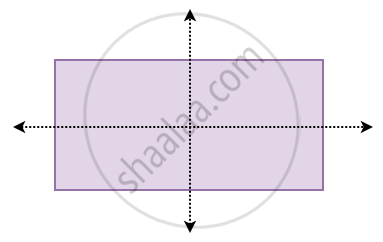#### Construction of figure with two Lines of Symmetry:

We can also learn to construct figures with two lines of symmetry starting from a small part.

1. Let us have a figure as shown alongside.2. We want to complete it so that we get a figure with two lines of symmetry. Let the two lines of symmetry be L and M.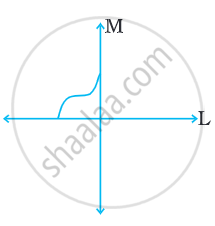3. We draw the part as shown to get a figure having line L as a line of symmetry.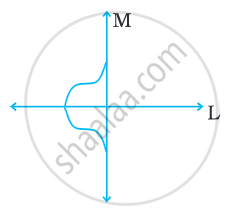4. To complete the figure we need it to be symmetrical about line M also. Draw the remaining part of the figure as shown. This figure has two lines of symmetry i.e. line Land line M.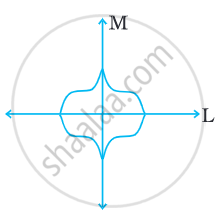#### 3. Figures with multiple Lines of Symmetry:

Example of multiple lines of symmetry (two or more)

• Take a square piece of paper. Fold it into half vertically, fold it again into half horizontally. (i.e. you have to fold it twice).
• Now open out the folds and again fold the square into half (for a third time now), but this time along a diagonal.
• Again open it and fold it into half (for the fourth time), but this time along the other diagonal.
• When you will open the paper you will see four imaginary lines and these lines are the lines of symmetry.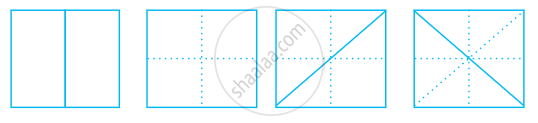### Some more images with more than two lines of symmetry: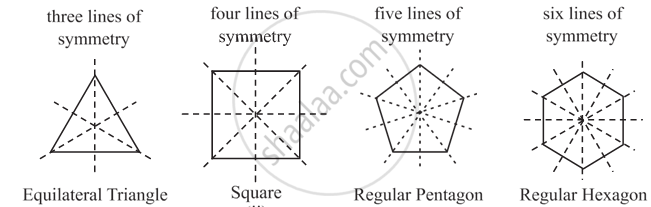• The equilateral triangle will have three lines of symmetry.

• Square will have four lines of symmetry.

• The regular pentagon will have five lines of symmetry.

• Regular hexagon will have six lines of symmetry.

If you would like to contribute notes or other learning material, please submit them using the button below.

### Shaalaa.com

Figures Having Two Or Multiple Lines Of Symmetry Part-1 [00:13:50]
S
0%